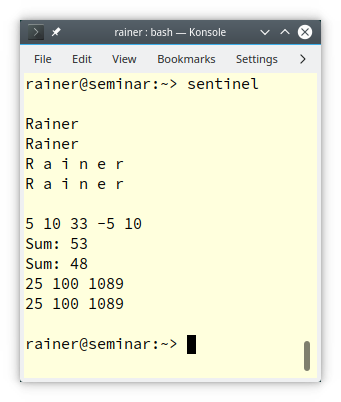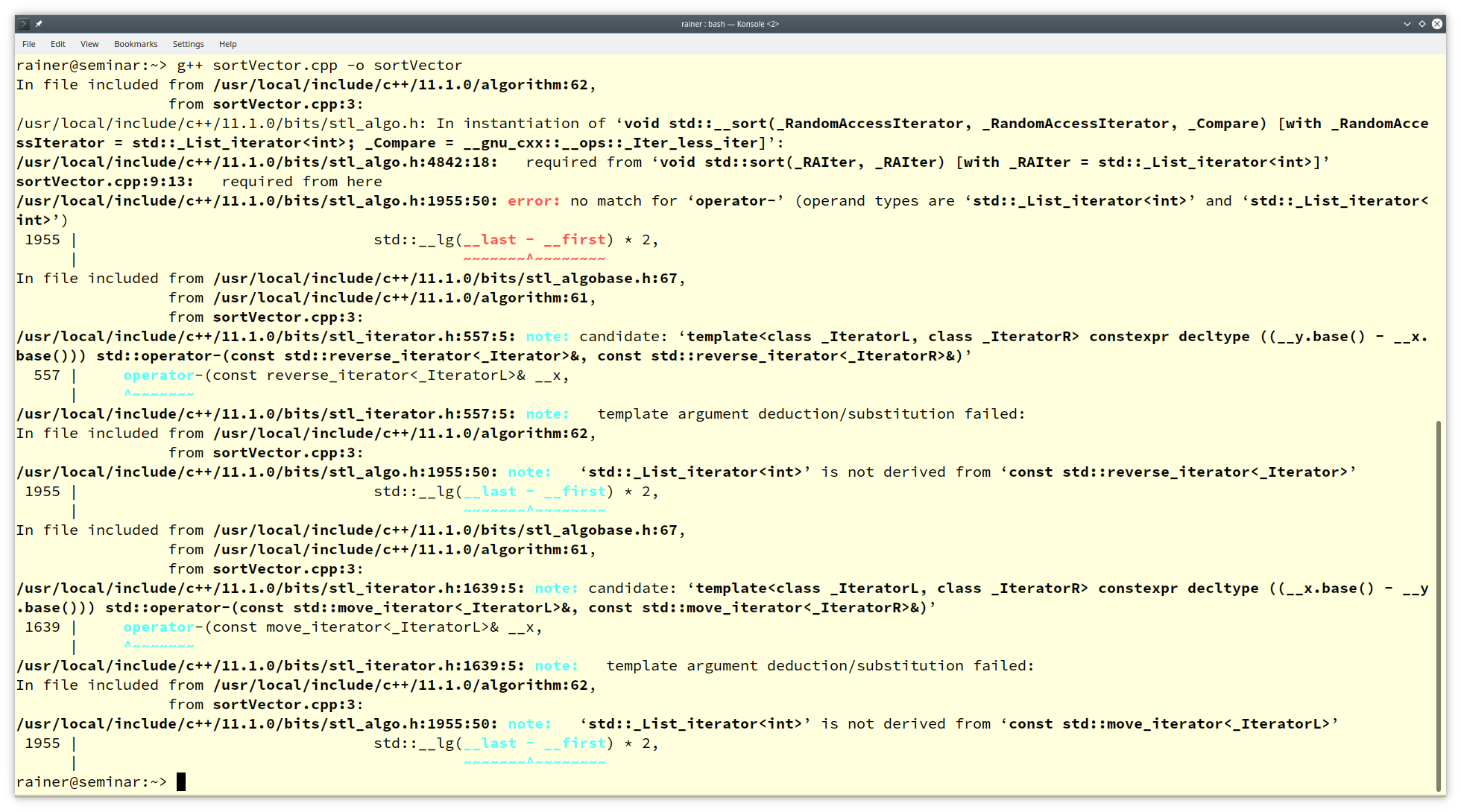# Sentinels and Concepts with Ranges Algorithms

The ranges library in C++20 supports sentinels. Sentinels stand for the end of a range and can be regarded as generalized end iterators.A range provided by a begin iterator and an end sentinel specifies a group of items you can iterate over. The containers of the STL are ranges because their end iterator marks the end of the range.

## Sentinel

The following example uses sentinels for a C-string and a `std::vector<int>`.

```// sentinel.cpp

#include <iostream>
#include <algorithm>
#include <compare>
#include <vector>

struct Space {                        // (1)
bool operator== (auto pos) const {
return *pos == ' ';
}
};

struct NegativeNumber {               // (2)
bool operator== (auto num) const {
return *num < 0;
}
};

struct Sum {                          // (7)
void operator()(auto n) { sum += n; }
int sum{0};
};

int main() {

std::cout << '\n';

const char* rainerGrimm = "Rainer Grimm";

std::ranges::for_each(rainerGrimm, Space{}, [] (char c) { std::cout << c; });  // (3)
std::cout << '\n';
for (auto c: std::ranges::subrange{rainerGrimm, Space{}}) std::cout << c;      // (4)
std::cout << '\n';

std::ranges::subrange rainer{rainerGrimm, Space{}};                            // (5)
std::ranges::for_each(rainer, [] (char c) { std::cout << c << ' '; });         // (6)
std::cout << '\n';
for (auto c: rainer) std::cout << c << ' ';
std::cout << '\n';

std::cout << "\n";

std::vector<int> myVec{5, 10, 33, -5, 10};

for (auto v: myVec) std::cout << v << " ";
std::cout << '\n';

auto [tmp1, sum] = std::ranges::for_each(myVec, Sum{});
std::cout << "Sum: " << sum.sum << '\n';                                   // (8)

auto [tmp2, sum2] = std::ranges::for_each(std::begin(myVec), NegativeNumber{},
Sum{} );
std::cout << "Sum: " << sum2.sum << '\n';                                   // (9)

std::ranges::transform(std::begin(myVec), NegativeNumber{},                 // (10)
std::begin(myVec), [](auto num) { return num * num; });
std::ranges::for_each(std::begin(myVec), NegativeNumber{},                  // (11)
[](int num) { std::cout << num << " "; });
std::cout << '\n';
for (auto v: std::ranges::subrange{ std::begin(myVec), NegativeNumber{}}) { // (12)
std::cout << v << " ";
}

std::cout << "\n\n";

}
```

The program defines two sentinels: Space (line 1) and `NegativeNumber` (line 2). Both define the equal operator. Thanks to the `<compare>` header, the compiler auto-generates the non-equal operator. The non-equal operator is required when using algorithms such as `std::ranges_for_each` or `std::ranges::transform` with a sentinel. Let me start with the sentinel `Space`.

Line (3) applies the sentinel `Space{}` directly onto the string “`rainerGrimm`“. Creating a `std::ranges::subrange` (line 4) allows it to use the sentinel in a range-based for-loop. You can also define a `std::ranges::subrange` and use it directly in the algorithm std::ranges::for_each (line 5) or in a range-based for-loop (line 6).

##Modernes C++ Mentoring

Be part of my mentoring programs:

• "Fundamentals for C++ Professionals" (open)
• "Design Patterns and Architectural Patterns with C++" (open)
• "C++20: Get the Details" (reopens December 2023)
• Do you want to stay informed about my mentoring programs: Subscribe via E-Mail.

My second example uses a `std::vector<int>`, filled with the values `{5, 10, 33, -5, 10}`. The sentinel `NegativeNumber` checks if a number is negative. First, I sum up all values using the function object `Sum` (line 7). `std::ranges::for_each` returns a pair `(it, func)``it` is the successor of the sentinel and `func` the function object applied to the range. Thanks to the structured binding, I can directly define the variables `sum `and `sum2 `and display their values (lines 8 and 9).` std::ranges::for_each` uses the sentinel `NegativeNumber`. Consequently, `sum2` has the sum up to the sentinel. The call `std::ranges::transform` (line 10) transforms each element to its square:` [](auto num){ return num * num}`. The transformation stops with the sentinel `NegativeNumber.` Line 11 and line 12 display the transformed values.

Finally, here is the output of the program.You may ask yourself, should I use a classical algorithm of the STL or the ranges pendant on a container? Let me answer this question by comparing both.

## `std` Algorithms versus `std::ranges` Algorithms

Before I dive into the details in my comparison, I want to provide the big picture:### Range does not support numeric

The ranges does support the functions of the `functional`, and the `algorithm `header, but the function of the `numeric` header.  `The numeric header includes `mathematical functions such as  `std::gcd, std::midpoint, std::iota,` or `std::accumulate.`

Let me write about more interesting differences.

### Concept support

The `std::ranges` algorithms are the poster child for concepts.

Let’s start with a comparison of the classical `std::sort` and the new` std::ranges::sort`. `std::sort` and `std::ranges::sort` require a random-access iterator that can access each range element in constant time. Here are the two relevant overloads for `std::sort` and `std::ranges::sort`.

• `std::sort`

```template< class RandomIt >
constexpr void sort( RandomIt first, RandomIt last );
```

• `std:ranges::sort`

```template <std::random_access_iterator I, std::sentinel_for<I> S,
class Comp = ranges::less, class Proj = std::identity>
requires std::sortable<I, Comp, Proj>
constexpr I sort(I first, S last, Comp comp = {}, Proj proj = {});
```

What happens when you invoke `std::sort` or `std::ranges::sort` with a container such as` std::list` only supporting a bidirectional iterator?

#### `std::sort`

```// sortVector.cpp

#include <algorithm>
#include <list>

int main() {

std::list<int> myList{1, -5, 10, 20, 0};
std::sort(myList.begin(), myList.end());

}
```

Compiling the program `sortVector.cpp` with the GCC causes an epic error message of 1090 lines.#### `std::ranges::sort`

```// sortRangesVector.cpp

#include <algorithm>
#include <list>

int main() {

std::list<int> myList{1, -5, 10, 20, 0};
std::ranges::sort(myList.begin(), myList.end());

}
```

Using` std::ranges::sort` instead of` std::sort reduces` the error message drastically. Now, I get 57 error lines.Honestly, the error message of GCC should be easier to read, but I don’t blame them. We are still in the early process of supporting concepts. Here are the first 10 lines of the 57 lines. I marked the critical message in red.## Which mentoring program should I implement next?

I’m happy that the current mentoring program, “Fundamentals for C++ Professionals”, is a big success and has more than 35 participants. Now, I will implement an additional mentoring program. All of them are based on my C++ books, posts, and classes.

## What’s next?

I’m not done with my comparison of  `std` and `std::ranges` algorithms. In my next post, I will write about the unified lookup rules that `std::ranges` algorithms provide and additional safety guarantees.

Thanks a lot to my Patreon Supporters: Matt Braun, Roman Postanciuc, Tobias Zindl, G Prvulovic, Reinhold Dröge, Abernitzke, Frank Grimm, Sakib, Broeserl, António Pina, Sergey Agafyin, Андрей Бурмистров, Jake, GS, Lawton Shoemake, Jozo Leko, John Breland, Venkat Nandam, Jose Francisco, Douglas Tinkham, Kuchlong Kuchlong, Robert Blanch, Truels Wissneth, Kris Kafka, Mario Luoni, Friedrich Huber, lennonli, Pramod Tikare Muralidhara, Peter Ware, Daniel Hufschläger, Alessandro Pezzato, Bob Perry, Satish Vangipuram, Andi Ireland, Richard Ohnemus, Michael Dunsky, Leo Goodstadt, John Wiederhirn, Yacob Cohen-Arazi, Florian Tischler, Robin Furness, Michael Young, Holger Detering, Bernd Mühlhaus, Matthieu Bolt, Stephen Kelley, Kyle Dean, Tusar Palauri, Dmitry Farberov, Juan Dent, George Liao, Daniel Ceperley, Jon T Hess, Stephen Totten, Wolfgang Fütterer, Matthias Grün, Phillip Diekmann, Ben Atakora, Ann Shatoff, Rob North, and Bhavith C Achar.

Thanks, in particular, to Jon Hess, Lakshman, Christian Wittenhorst, Sherhy Pyton, Dendi Suhubdy, Sudhakar Belagurusamy, Richard Sargeant, Rusty Fleming, John Nebel, Mipko, Alicja Kaminska, Slavko Radman, and David Poole.

 My special thanks to EmbarcaderoMy special thanks to PVS-StudioMy special thanks to Tipi.buildMy special thanks to Take Up Code## Seminars

I’m happy to give online seminars or face-to-face seminars worldwide. Please call me if you have any questions.

### Standard Seminars (English/German)

Here is a compilation of my standard seminars. These seminars are only meant to give you a first orientation.

• C++ – The Core Language
• C++ – The Standard Library
• C++ – Compact
• C++11 and C++14
• Concurrency with Modern C++
• Design Pattern and Architectural Pattern with C++
• Embedded Programming with Modern C++
• Generic Programming (Templates) with C++

#### New

• Clean Code with Modern C++
• C++20

### Contact Me

Modernes C++ Mentoring,Tags:
0 replies